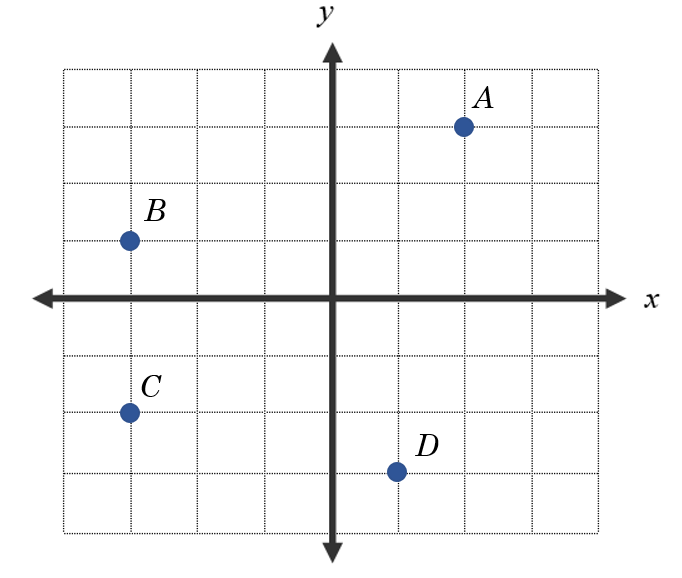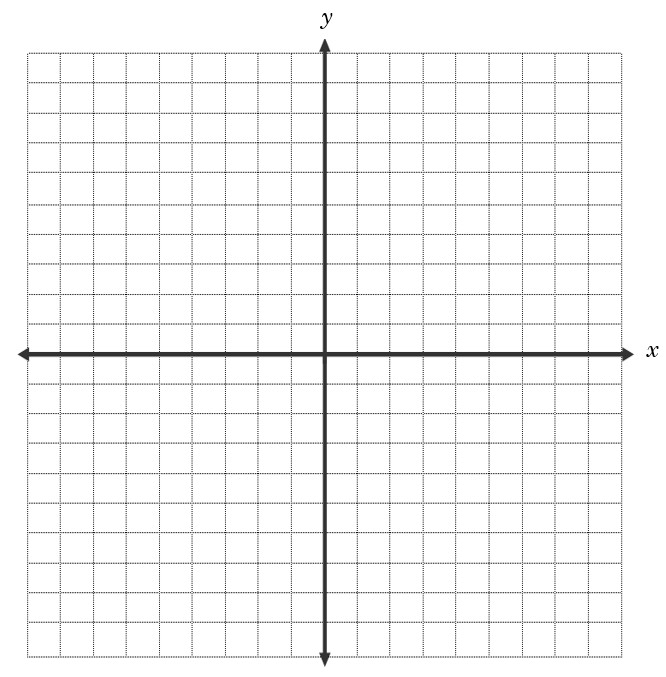# The Coordinate System

The system for graphing points in a plane known as the Cartesian coordinate system, was invented by French philosopher, mathematician, and scientist, René Descartes, in the 1600’s.  In this system, every point can be represented by an ordered pair $$(x, y)$$ where $$x$$ represents the horizontal location, and $$y$$ represents the vertical location.  These locations are measured from the intersection of the $$x$$-axis and $$y$$-axis, which is the point $$(0, 0)$$ and is called the origin.

1.  The graph below contains four ordered pairs.  Match each ordered pair to the appropriate point.$$(1, -3)$$: Point _ $$(-3, 1)$$: Point _ $$(-3, -2)$$: Point _ $$(2, 3)$$: Point _

The $$x$$-axis and the $$y$$-axis divide the graphing plane into four regions called Quadrants I, II, III, and IV.  They are numbered counter-clockwise, starting with the upper right quadrant, as shown below.  Points that lie on an axis do not lie in any quadrant.

2.  Name the appropriate quadrant for each point below.$$(-2, -1)$$ lies in Quadrant _. $$(2, -1)$$ lies in Quadrant _. $$(-2, -1)$$ lies in Quadrant _. $$(2, 1)$$ lies in Quadrant _.

3.  Graph and label the following points on the coordinate axes below, then connect the points with line segments in alphabetical order.$$A(5, -3)$$ $$B(-6, 8)$$ $$C(-2, -4)$$ $$D(0, -10)$$ $$E(2, -4)$$ $$F(5, 5)$$ $$G(-7, -1)$$ $$H(5, -3)$$

4.  Which quadrant contains the most points?

Check solutions here.